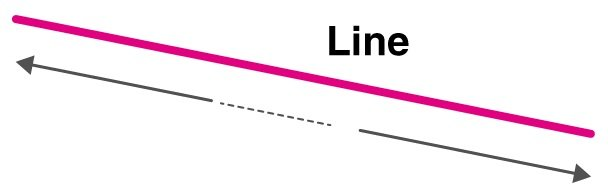# Common Concept of Lines but on a Different Level

In mathematics, there is a particular topic which we have been studying for our first class. The base is the same but, it increases its difficulty level in higher-level studies. Students commonly come across this topic in every position, either a chapter or a part of other topics. If not necessary, they also get some glimpses of this topic. Here we are talking about the concept of lines.

Straight lines are the most common lines which are being use in mathematical concepts and an example of this is the number line. Many people get confused in the line that is endless or has some limit points. A straight line is endless, which means that it has no limit points. That is why its ends are depicted as arrows to shows that the line is endless.

This topic of straight lines is being studied at higher-level, where it has different types, formulas, and equations. Many students find that topic a little bit complex and confusing as it is being studied at a higher level.## Here are some tips for those students who are finding difficulty in this particular concept:

### 1. Start from the basics

If the student has no confidence in attempting the questions, they will start choosing random examples and try to solve them. But this is the wrong method to do so. Students should start by solving first-level questions in their subject book and easy once is to first solve the solved examples. That will give them a basic idea about the concept. If they become strong at that point, they can move forward to a higher level.

### 2. Formula sheet

Many people think that making a proper sheet for the various formulas is a bad idea, but, it is very helpful for the students to use them while solving the starting questions and eventually, they will remember all the formulas. They can use all these formulas in solving the problems. But before making a sheet, they should first understand the equations and formulas step by step and should have in-depth information about the whole concept. If they do not want to stop while solving any problem, they can take help of this sheet but firstly they should try to remember the formula and use it. If they fail to do that then they can take help from the formula sheet.

### 3. Practicing strategy

Students should make a whole strategy for solving the various questions and clearing their doubts. They should not stop by solving 1-2 questions, this will not help them in learning the whole concept. This chapter comes with different questions and concepts. A person should at least solve 10-15 questions per day to become strong in the concept. They should practice the previous year’s questions if they have completed the base book and want to go for higher-level concepts. To keep their grip on the concept. They should not get frustrated with their answers are not correct, they should keep solving till they get the concept clarity.

From the points mentioned above, we can say that learning about the straight line is interesting and fun. People should try all types of questions related to this concept. They should search for some introductory books to get more information about the concept. Students should keep that in mind that other lines such as perpendicular lines come under the same category of lines but they are different from each other. If students want to get more detailed information about the concept of straight lines, they can get that from the Cuemath website. This website has experts to solve any type of problem students and facing and also give an in-depth about every concept.

The concept of straight lines can be understood properly with the help of these experts. People should try to learn from this concept as it is a very common topic but with the time being, its formulas and equations have got a level up.